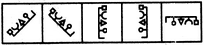# Non Verbal Reasoning - Classification - Discussion

### Discussion :: Classification - Section 1 (Q.No.28)

In each problem, out of the five figures marked (1), (2), (3), (4) and (5), four are similar in a certain manner. However, one figure is not like the other four. Choose the figure which is different from the rest.

28.

Choose the figure which is different from the rest.(1)     (2)     (3)     (4)     (5)

 [A]. 1 [B]. 2 [C]. 3 [D]. 4 [E]. 5

Explanation:

All other figures can be rotated into each other.

 Surya said: (Mar 18, 2011) Allother rotated into each other - what does it mean?. Please give me more explanation.

 Nagendra said: (Jun 27, 2011) If you rotate the given figure. You will get any one figure among the four except 4th one. See take the 1st figure and rotate it 90 degree CW degree you will get 2nd figure. If rotate the 1st figure 45 degree ACW you will get 3th figure. If you still rotate ACW or CW you will get last figure. But you will not get the 4th figure because it's a mirror image.

 Barbie said: (Jul 2, 2011) thanq nagendra.

 Arunn said: (Sep 24, 2015) I seriously thought that figure three was square, semi-circle, triangle, SQUARE and therefore not the same as the other four figures.

 Akshay said: (Feb 18, 2017) I Think Option 3 is the correct Answer because in this pic. There are 2 square in other there are only single diagram of any fig.

 Aastha said: (Apr 7, 2017) I think the answer should be C, as the shapes are following a particular pattern.

 Shagun said: (Jun 6, 2017) I can't understand. Please tell me clearly.

 Keerthikumari O C said: (Feb 23, 2021) Option C is the correct answer because all other diagrams there is a circle after the triangle but in option C, it is in square.

 Anonde Bennett said: (Jun 17, 2021) The correct answer is option C. Because The pin (it's head) in option is a quadrilateral whereas others are circular.